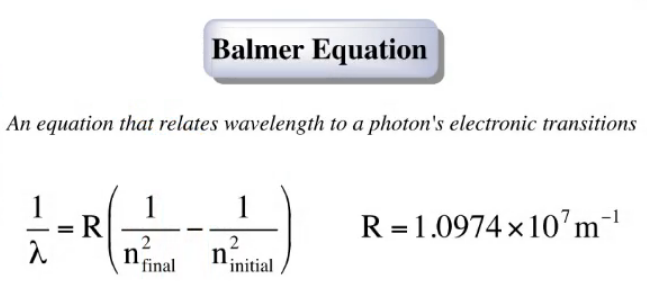# Problem: Does a photon of visible light (λ ≈ 400 to 700 nm) have sufficient energy to excite an electron in a hydrogen atom from the n = 1 to the n = 5 energy state? From the n = 2 to the n = 6 energy state?

###### FREE Expert Solution

We use the Balmer Equation below to get the wavelength, needed to excite an electron with the given situations.We have to know that this equation is written to provide the wavelength for the energy emitted when an electron goes from a higher to a lower energy level.   To get the right sign, we just need to reverse the final and initial energy levels.

81% (115 ratings)###### Problem Details

Does a photon of visible light (λ ≈ 400 to 700 nm) have sufficient energy to excite an electron in a hydrogen atom from the n = 1 to the n = 5 energy state? From the n = 2 to the n = 6 energy state?